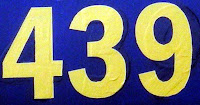## Friday, July 2, 2010

### 439

439 is a prime number.

439 is the sum of three consecutive primes (439 = 139 + 149 + 151) and the sum of nine consecutive primes (439 = 31 + 37 + 41 + 43 + 47 + 53 + 59 + 61 + 67).

439 is a number that can be expressed as the signed sum of cubes in at least three ways: 439 = 2644883 + 3315743 - 3801933 = 23224043 - 8694183 - 22810573 = 4029063 - 2114023 - 3824893.

439 is the smallest prime such that another prime is never produced by inserting the same digit between each pair of its digits: 40309, 41319, 42329, . . . 49399 are all composite.

439 is a number that cannot be written as a sum of three squares.439 Ohio is a main-belt asteroid discovered in 1898.

#### 1 comment:Anonymous said...

439=2^9-73
439=21^2-2 es un primo de la forma n^2-2
439 es un primo que sigue siendo primo 43(9) -> 43 si desaparece el numero de la derecha
439^2=96361^2-96360^2
439 se puede representar como diferencia de numeros con exponente 7:
439^7=568^7-(127^7+258^7+266^7+413^2+430^7+525^7)
Rafael Parra de Barcelona